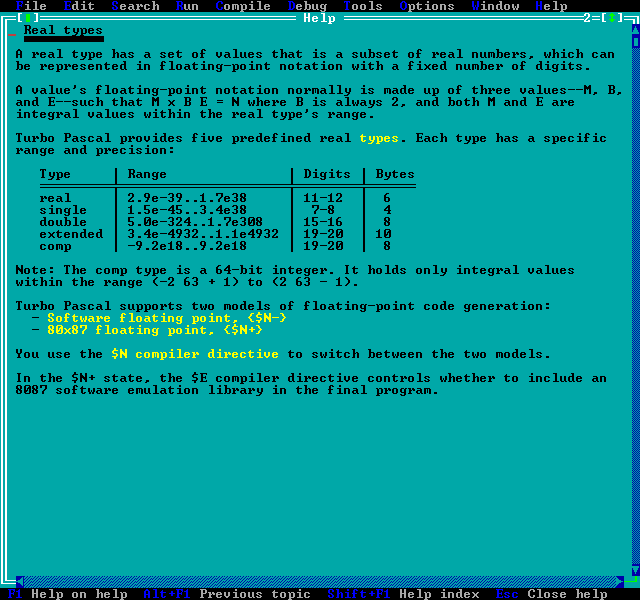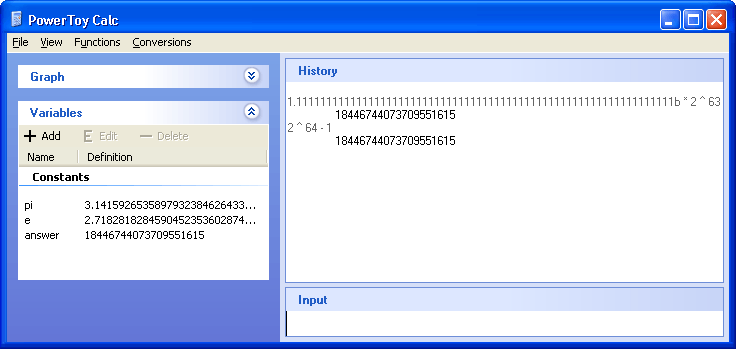Turbo Pascal's 64-bit comp Type

Saturday, July 29, 2006
By: Jason Doucette

Back in the day, the comp data type in Turbo Pascal, a 16-bit compiler (which compiled 8-bit code by default), allowed you to store 64-bit signed integer values.  But it was a very strange type.  It was not considered an integer.  It was considered a 'real' (floating point) type by the language:You could not increment or decrement it with the normal integer increment decrement functions, Inc() and Dec() (the equivalent to the postfix increment and decrement operators in C / C++: ++ and --).  You had to deal with comp as a floating point value in computations.  Thus, the following is required to increment / decrement it: x = x + 1.0; and  x = x - 1.0;  If you printed the value to the screen with WriteLn() it would be displayed as a floating point value.  It also had a curious range of -(2^63)+1 to +(2^63)-1 (-9,223,372,036,854,775,807 to 9,223,372,036,854,775,807) such that the magnitude of the lower and upper bounds are equal.  We would expect an 8-byte signed integer to have the range of -2^63 to +2^63-1 (-9,223,372,036,854,775,808 to 9,223,372,036,854,775,807), just as a 2-byte signed integer would store -32,768 to 32,767 (there is one less possible positive value than there are negative values because zero is stored in the 'positive half').  Who concocted such a strange, mongrel data type?

Why Is comp Considered A Floating Point?

The key lies in how floating point values are stored.  A floating point number stores three components: a sign, an exponent, and a mantissa.  The value that the floating point bits represent is as follows:

value = sign * mantissa * 2 ^ exponent

• sign = -1 or +1.  This is stored in one bit.

• mantissa (also known as the significand) = a value from 1.0 to 2.0, but cannot be exactly 2.0:  1.0 <= mantissa < 2.0.  It is stored in 23 bits for a IEEE 32-bit floating point, 52 bits for a IEEE 64-bit floating point, 64-bits for a IEEE 80-bit floating point.  Since all values 1.0 <= x < 2.0 stored in binary have the first bit as a 1 (1.0 in binary = 1.000..., and 1.999... in binary = 1.111...), it is not stored, but implied.  Such storage is known as 'packed'.  Therefore, the precision of the mantissa is actually one larger than its storage size.  The FPU internals have to 'unpack' the number, by inserting the missing bit, to make use of it.  Note that the 80-bit type does not have a packed type, since the FPU's internal registers do not have enough space to store the extra bit if there were one.

• exponent = an integer value that stores positive and negative exponents.  It is stored in 8 bits for a IEEE 32-bit floating point (representing -126 to 127), 11 bits for a IEEE 64-bit floating point (representing -1022 to 1023), 15-bits for a IEEE 80-bit floating point (representing -16,382 to 16,383).  (Note that two possible combinations of these bits are used to represent irregular numbers, such as infinity, NaN (Not a Number), and zero.)

What this implies, but may not be immediately obvious, is that many integers can be stored with full accuracy in a floating point representation.  Any integer that does not require more precision than what the mantissa can store can be represented exactly.  The IEEE 80-bit floating point type stores 64-bits, plus the implied 1 bit, for a total of 65-bits for the mantissa.  Thus, it could can all possible 64-bit integer values.  So, was the comp type actually an 80-bit floating point type?

This would explain the strange range limits of -(2^63)+1 to +(2^63)-1 (-9,223,372,036,854,775,807 to 9,223,372,036,854,775,807), since floating point values store the sign bit separately from the magnitude data.  Thus, the smallest possible negative value always has the same magnitude as the largest possible positive value.

How Large Is comp?

But, if it were an 80-bit floating point type, it would take up 10 bytes of memory, instead of only 8.  I have Turbo Pascal kicking around on my hard drive, so let's see if it does.  I made the following program:

program comp_type;
var
x: comp;
a: array[1..1000] of comp;

begin
x := 1.0;
writeln('x = ',x);
writeln('sizeof(x) = ',sizeof(x),' bytes.');
writeln('sizeof(a) = ',sizeof(a),' bytes.');
end.

This produces the following output:

x =  1.00000000000000E+0000
sizeof(x) = 8 bytes.
sizeof(a) = 8000 bytes.

Hmm... so it is only stored in 8 bytes, not 10.  Well, this makes sense.  If we are only ever storing 64-bits of information, we should only use 64 bits to store it in, not 80 bits.  When the time is needed to perform computations on this data, we can convert it into the 80-bit floating point type.  So, using comp means it would be slower than expected due to this.  But, I guess this should come at no surprise, considering you cannot use normal integer operations on the type.  After all, the comp type is defined as a real (i.e. floating point type) in the Turbo Pascal manuals.

What Integers Can 80-bit Floating Point Store?

The largest fully represented integer (such that every bit of the integer is accounted for by the mantissa) the 80-bit floating point type can store has the following attributes:  The mantissa, in binary, is composed entirely with 1's.  Recalling that there is no implied 1 bit for the 80-bit floating point, this dictates 64 1's, not 65.  And the exponent is just high enough such that the last bit of the mantissa represents the least significant bit of the integer (the 1's place) we are trying to represent.  This state occurs when the exponent = 63.  So, we have:

1.111111111111111111111111111111111111111111111111111111111111111b * 2 ^ 63 = 18,446,744,073,709,551,615

There should be exactly 64 1's in the first number above.  The postfix "b" means the number is binary.  Without the "b", the number is decimal.  The result is shown in decimal.  You can type this equation, exactly as-is (everything before the equals sign) into Microsoft's PowerToy Calculator.  Make sure you have the precision set high enough so the program will maintain enough digits of accuracy, as "Low Precision (32)" is not enough.  You can change it by going into the View menu -> Advanced Options.  I recommend "High Precision (128)", since "Medium Precision (64)" is just barely at the limit of the accuracy we need.Why Can't comp Store -2^63?

The result above, 18,446,744,073,709,551,615, is equivalent to 2^64-1.  Thus, the 80-bit floating point format can store integers in full accuracy from -2^64-1 to +2^64-1.  This is more than enough to store the standard range of a normal signed 64-bit integer (-2^63 to +2^63-1).  So, why does comp only store from -(2^63)+1 to 2^63-1?  What is going on here?

I have no idea.  Let's try and force it to be stored with a Turbo Pascal program.  The following program sets a comp data type to be 2^63-1, which is the maximum signed 64-bit integer which comp can store.  It negates it, and stores -2^63+1, which is supposedly the lowest value comp can store, but it one higher than the smallest value a signed 64-bit integer can store.  We then subtract one from this to get -2^63, and see what happens...

program comp_type_2;
var
x: comp;
e: extended;
i: integer;

procedure showbits(x: comp);
var
pb: ^byte;
i,j: integer;
begin
pb := @x;
inc(pb,7);
for i := 7 downto 0 do
begin
for j := 7 downto 0 do
write(ord(pb^ and (1 shl j) <> 0));
dec(pb);
end;
writeln;
end;

begin
e := 1.0;
for i := 1 to 63 do
e := e * 2.0;
e := e - 1.0; {e = 2^63 - 1 = maximum signed 64-bit integer}
x := e; {2^63-1}
showbits(x);
x := -e; {-2^63+1}
showbits(x);
x := x - 1.0; {-2^63}
showbits(x);
{x := x - 1.0; {uncomment to cause: Error 207: Invalid floating point operation}
end.

The output is as follows, which clearly indicates that the values are stored in two's complement format, as are almost all integers:

0111111111111111111111111111111111111111111111111111111111111111 (+2^63-1)
1000000000000000000000000000000000000000000000000000000000000001 (-2^63+1)
1000000000000000000000000000000000000000000000000000000000000000 (-2^63)

comp could store -2^63, despite the restriction mentioned in the Turbo Pascal help file.  To ensure this is the case, if you uncomment out the second subtraction, it will reveal an error: "Error 207: Invalid floating point operation"

I think the original design of comp was made knowing that floating point values can store both negative and positive values of the same magnitude, and it was thought this would be a restriction in the comp storage.  It was unknown at this time that the 80-bit floating point type could easily store all possible signed 64-bit integer values, and this mistake continued forward into the Turbo Pascal help file.  It is just a simple error.

• Excerpt from the Turbo Pascal manual:

Comp     -2^63+1 to 2^63-1     8 bytes

\$N+, \$E+ required for use.  Provides 19 to 20 digits of accuracy in a 64 bit Longint-type.  The values range from -9.2 x 10^18 to 9.2 x 10^18.  Comp types are used for integer values only, but are implemented in the math coprocessor and the 8087 floating point software emulation routines.

• Intel 80x87 Floating Point Data Types

Website corrections:

The "smallest positive denormal" listings are all incorrect (for 32-bit, 64-bit, and 80-bit).  They were calculated incorrectly as the author assumed that an exponent stored as 0 internally is one smaller than an exponent stored as 1 internally.  However, this is not true.  An exponent stored as 0 internally is a special case which indicates the mantissa is 'unpacked' and does not have an implied first 1 bit that is not stored (for 80-bit numbers, this is always true, actually).  But, in this case, the exponent of value 0 still represents the smallest exponent value possible (-126 for 32-bit, -1,022 for 64-bit, and-16,382 for 80-bit).

The web page states that the "largest fp integer with a predecessor" listing is claimed to be "The largest floating point integer N such that N-1 is also a floating point integer".  However, if this value is N, you will notice that N + 1 is also an integer, and N + 1 has a predecessor, N.  So, the description is incorrect.  The numbe N shown is correct, but is not described properly.  It is, as I described it in the above article, the largest fully represented integer (such that every bit of the integer is accounted for by the mantissa).

About the Author: I am Jason Doucette of Xona Games, an award-winning indie game studio that I founded with my twin brother. We make intensified arcade-style retro games. Our business, our games, our technology, and we as competitive gamers have won prestigious awards and received worldwide press. Our business has won \$180,000 in contests. Our games have ranked from #1 in Canada to #1 in Japan, have become #1 best sellers in multiple countries, have won game contests, and have held 3 of the top 5 rated spots in Japan of all Xbox LIVE indie games. Our game engines have been awarded for technical excellence. And we, the developers, have placed #1 in competitive gaming competitions -- relating to the games we make. Read about our story, our awards, our games, and view our blog.